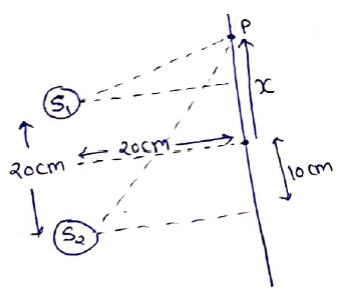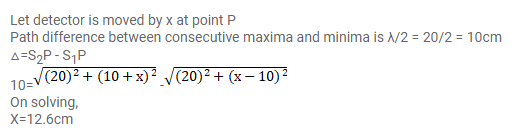# The two sources of sound, S1 and S2,Question:

The two sources of sound, $S_{1}$ and $S_{2}$, emitting waves of equal wavelength $20.0 \mathrm{~cm}$, are placed with a separation of $20.0$ cm between them. A detector can be moved on a line parallel to $S_{1} S_{2}$ and at a distance of $20.0 \mathrm{~cm}$ from it. Initially, the detector is equidistant from the two sources. Assuming that the waves emitted by the sources are in detector should be shifted to detect a minimum of sound.

Solution: